# Scale Delta t VI

## LabVIEW 2018 Help

Edition Date: March 2018
Part Number: 371361R-01
View Product InfoLabVIEW 2016 HelpLabVIEW 2017 HelpLabVIEW 2018 HelpLabVIEW 2019 HelpLabVIEW 2020 Help

Owning Palette: Waveform VIs and Functions

Requires: Base Development System

Multiplies the delta t component of the waveform by the specified scale factor. Generally, this lengthens or shortens the sample rate of the waveform. The data type you wire to the waveform in input and the data type of the Y component of the analog waveform determine the polymorphic instance to use.

Use the pull-down menu to select an instance of this VI.

 Select an instance WDT Scale Delta t DBLWDT Scale Delta t SGLWDT Scale Delta t I64WDT Scale Delta t I32WDT Scale Delta t I16WDT Scale Delta t CDBWDT Scale Delta t EXTDWDT Scale Delta t

## WDT Scale Delta t DBL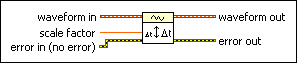waveform in is the waveform for which you want to scale the delta t. The default is empty.scale factor is the number by which you multiply the waveform's delta t. A value of greater than zero but less than 1 shortens the sample rate. A value of greater than 1 increases the sample rate. The default is 1000.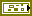error in describes error conditions that occur before this node runs. This input provides standard error in functionality.waveform out returns the resulting waveform with the new delta t value.error out contains error information. This output provides standard error out functionality.

## WDT Scale Delta t SGLwaveform in is the waveform for which you want to scale the delta t. The default is empty.scale factor is the number by which you multiply the waveform's delta t. A value of greater than zero but less than 1 shortens the sample rate. A value of greater than 1 increases the sample rate. The default is 1000.error in describes error conditions that occur before this node runs. This input provides standard error in functionality.waveform out returns the resulting waveform with the new delta t value.error out contains error information. This output provides standard error out functionality.

## WDT Scale Delta t I64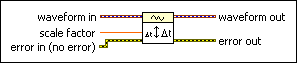waveform in is the waveform for which you want to scale the delta t. The default is empty.scale factor is the number by which you multiply the waveform's delta t. A value of greater than zero but less than 1 shortens the sample rate. A value of greater than 1 increases the sample rate. The default is 1000.error in describes error conditions that occur before this node runs. This input provides standard error in functionality.waveform out returns the resulting waveform with the new delta t value.error out contains error information. This output provides standard error out functionality.

## WDT Scale Delta t I32waveform in is the waveform for which you want to scale the delta t. The default is empty.scale factor is the number by which you multiply the waveform's delta t. A value of greater than zero but less than 1 shortens the sample rate. A value of greater than 1 increases the sample rate. The default is 1000.error in describes error conditions that occur before this node runs. This input provides standard error in functionality.waveform out returns the resulting waveform with the new delta t value.error out contains error information. This output provides standard error out functionality.

## WDT Scale Delta t I16waveform in is the waveform for which you want to scale the delta t. The default is empty.scale factor is the number by which you multiply the waveform's delta t. A value of greater than zero but less than 1 shortens the sample rate. A value of greater than 1 increases the sample rate. The default is 1000.error in describes error conditions that occur before this node runs. This input provides standard error in functionality.waveform out returns the resulting waveform with the new delta t value.error out contains error information. This output provides standard error out functionality.

## WDT Scale Delta t CDBwaveform in is the waveform for which you want to scale the delta t. The default is empty.scale factor is the number by which you multiply the waveform's delta t. A value of greater than zero but less than 1 shortens the sample rate. A value of greater than 1 increases the sample rate. The default is 1000.error in describes error conditions that occur before this node runs. This input provides standard error in functionality.waveform out returns the resulting waveform with the new delta t value.error out contains error information. This output provides standard error out functionality.

## WDT Scale Delta t EXTwaveform in is the waveform for which you want to scale the delta t. The default is empty.scale factor is the number by which you multiply the waveform's delta t. A value of greater than zero but less than 1 shortens the sample rate. A value of greater than 1 increases the sample rate. The default is 1000.error in describes error conditions that occur before this node runs. This input provides standard error in functionality.waveform out returns the resulting waveform with the new delta t value.error out contains error information. This output provides standard error out functionality.

## DWDT Scale Delta t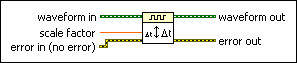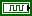waveform in is the waveform for which you want to scale the delta t. The default is empty.scale factor is the number by which you multiply the waveform's delta t. A value of greater than zero but less than 1 shortens the sample rate. A value of greater than 1 increases the sample rate. The default is 1000.error in describes error conditions that occur before this node runs. This input provides standard error in functionality.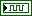waveform out returns the resulting waveform with the new delta t value.error out contains error information. This output provides standard error out functionality.